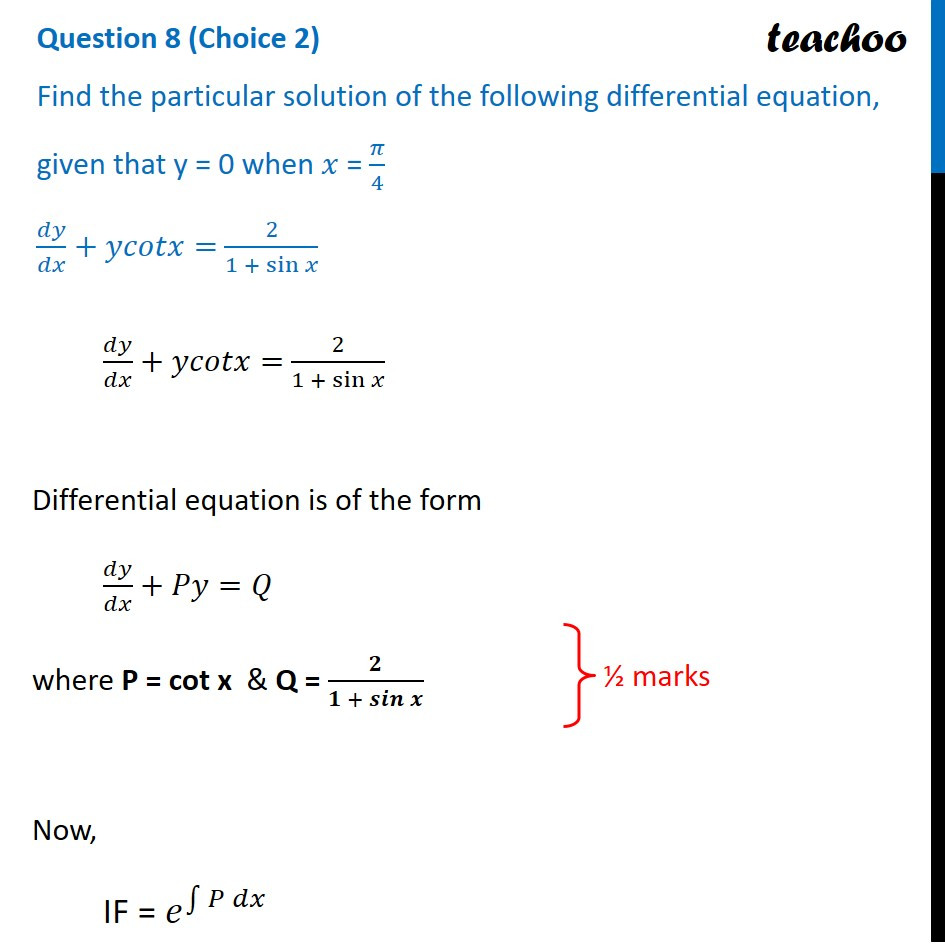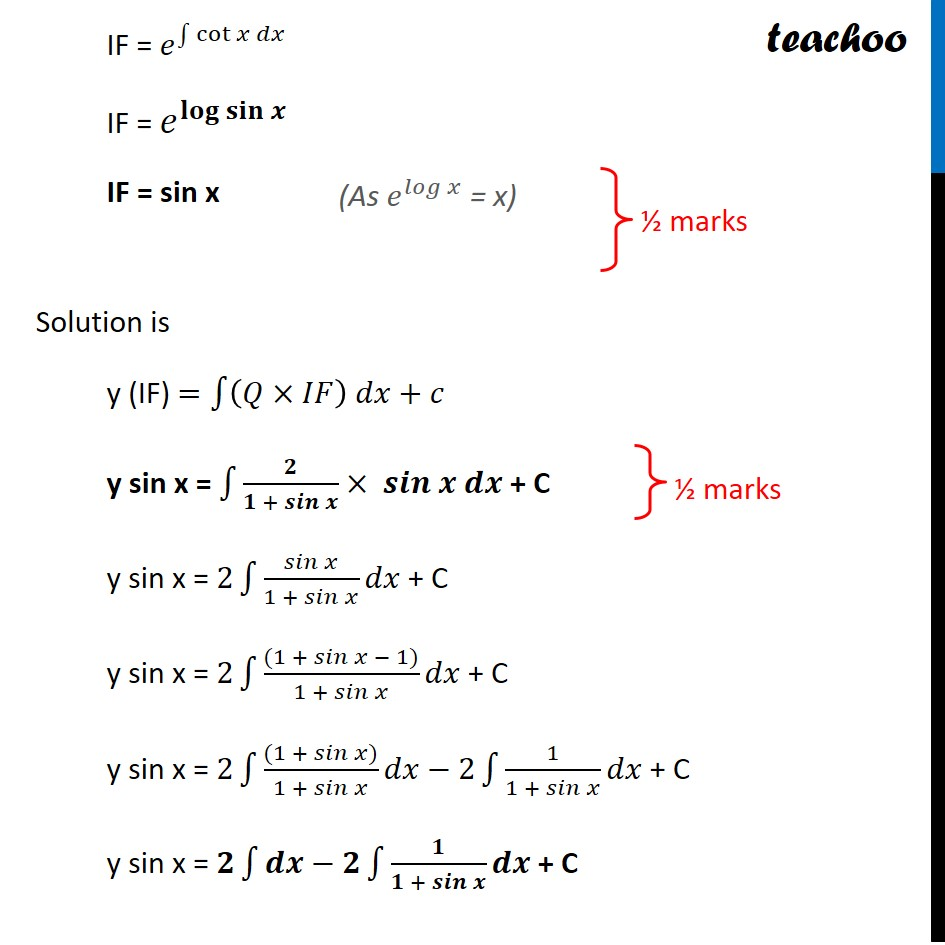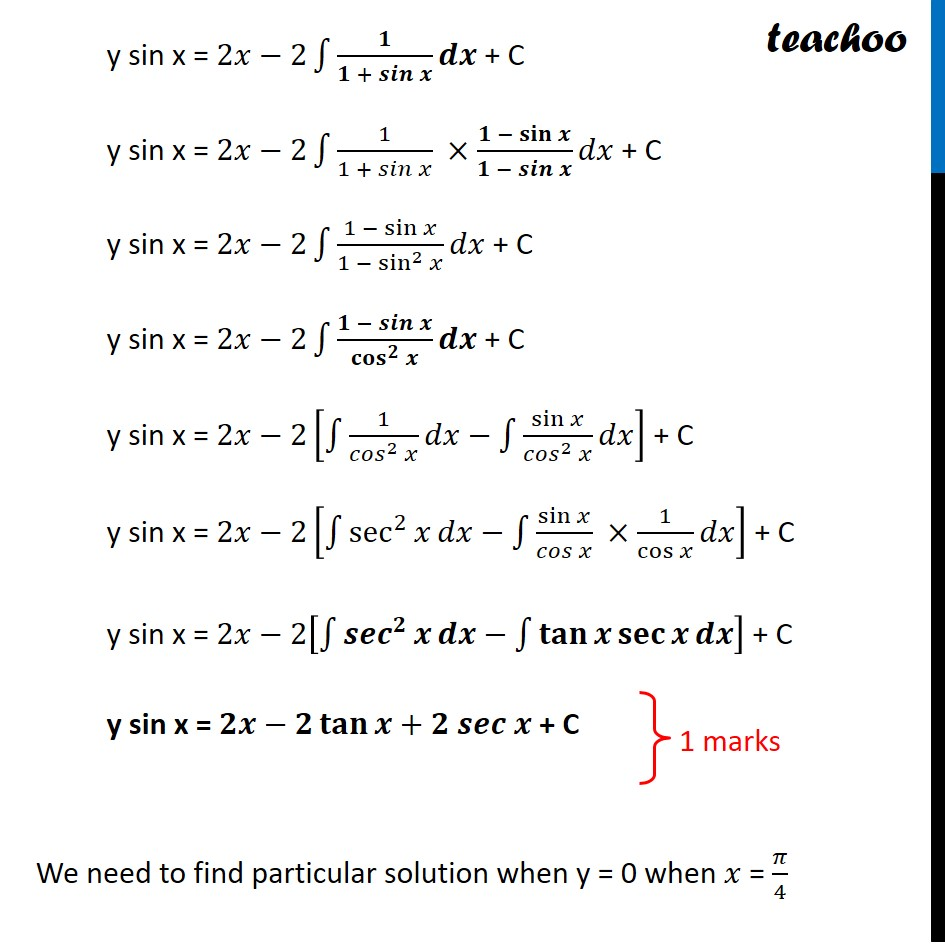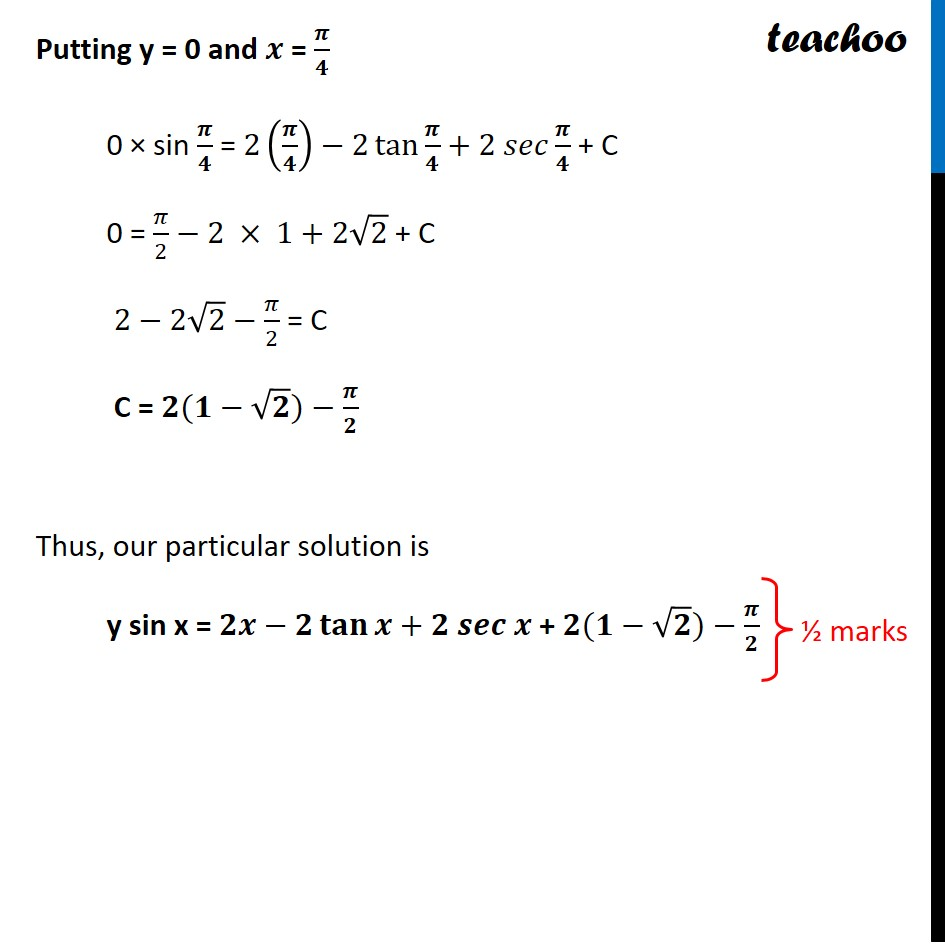CBSE Class 12 Sample Paper for 2022 Boards (For Term 2)

Class 12
Solutions of Sample Papers and Past Year Papers - for Class 12 Boards

## Find the particular solution of the following differential equation, given that y = 0 when 𝑥 = 𝜋/4 𝑑𝑦/𝑑𝑥+𝑦𝑐𝑜𝑡𝑥 2/(1 + sin⁡𝑥 )

This question is similar to Example 22 - Chapter 9 Class 12 - Differential EquationsGet live Maths 1-on-1 Classs - Class 6 to 12

### Transcript

Question 8 (Choice 2) Find the particular solution of the following differential equation, given that y = 0 when 𝑥 = 𝜋/4 𝑑𝑦/𝑑𝑥+𝑦𝑐𝑜𝑡𝑥= 2/(1 + sin⁡𝑥 ) 𝑑𝑦/𝑑𝑥+𝑦𝑐𝑜𝑡𝑥= 2/(1 + sin⁡𝑥 ) Differential equation is of the form 𝑑𝑦/𝑑𝑥+𝑃𝑦=𝑄 where P = cot x & Q = 𝟐/(𝟏 + 𝒔𝒊𝒏⁡𝒙 ) Now, IF = 𝑒^∫1▒〖𝑃 𝑑𝑥〗 IF = 𝑒^∫1▒〖cot⁡𝑥 𝑑𝑥〗 IF = 〖𝑒^𝐥𝐨𝐠⁡𝐬𝐢𝐧⁡𝒙 〗^" " IF = sin x Solution is y (IF) =∫1▒〖(𝑄×𝐼𝐹) 𝑑𝑥+𝑐〗 y sin x = ∫1▒〖𝟐/(𝟏 + 𝒔𝒊𝒏⁡𝒙 )× 𝒔𝒊𝒏 𝒙 𝒅𝒙〗 + C y sin x = 2∫1▒〖(𝑠𝑖𝑛 𝑥)/(1 + 𝑠𝑖𝑛⁡𝑥 ) 𝑑𝑥〗 + C y sin x = 2∫1▒〖((1 + 𝑠𝑖𝑛 𝑥 − 1))/(1 + 𝑠𝑖𝑛⁡𝑥 ) 𝑑𝑥〗 + C y sin x = 2∫1▒〖((1 + 𝑠𝑖𝑛 𝑥))/(1 + 𝑠𝑖𝑛⁡𝑥 ) 𝑑𝑥〗−2∫1▒〖1/(1 + 𝑠𝑖𝑛⁡𝑥 ) 𝑑𝑥〗 + C y sin x = 𝟐∫1▒𝒅𝒙−𝟐∫1▒〖𝟏/(𝟏 + 𝒔𝒊𝒏⁡𝒙 ) 𝒅𝒙〗 + C y sin x = 2𝑥−2∫1▒〖𝟏/(𝟏 + 𝒔𝒊𝒏⁡𝒙 ) 𝒅𝒙〗 + C y sin x = 2𝑥−2∫1▒〖1/(1 + 𝑠𝑖𝑛⁡𝑥 ) ×(𝟏 − 𝐬𝐢𝐧⁡𝒙)/(𝟏 − 𝒔𝒊𝒏⁡𝒙 ) 𝑑𝑥〗 + C y sin x = 2𝑥−2∫1▒〖(1 − sin⁡𝑥)/(1 − sin^2⁡𝑥 ) 𝑑𝑥〗 + C y sin x = 2𝑥−2∫1▒〖(𝟏 − 𝒔𝒊𝒏⁡𝒙)/〖𝐜𝐨𝐬〗^𝟐⁡𝒙 𝒅𝒙〗 + C y sin x = 2𝑥−2[∫1▒〖1/〖𝑐𝑜𝑠〗^2⁡𝑥 𝑑𝑥〗−∫1▒〖sin⁡𝑥/〖𝑐𝑜𝑠〗^2⁡𝑥 𝑑𝑥〗] + C y sin x = 2𝑥−2[∫1▒〖sec^2⁡𝑥 𝑑𝑥〗−∫1▒〖sin⁡𝑥/(𝑐𝑜𝑠 𝑥) ×1/cos⁡𝑥 𝑑𝑥〗] + C y sin x = 2𝑥−2[∫1▒〖〖𝒔𝒆𝒄〗^𝟐⁡𝒙 𝒅𝒙〗−∫1▒〖𝐭𝐚𝐧⁡𝒙 𝐬𝐞𝐜⁡𝒙 𝒅𝒙〗] + C y sin x = 𝟐𝒙−𝟐 𝐭𝐚𝐧⁡𝒙+𝟐 𝒔𝒆𝒄 𝒙 + C We need to find particular solution when y = 0 when 𝑥 = 𝜋/4 Putting y = 0 and 𝒙 = 𝝅/𝟒 0 × sin 𝝅/𝟒 = 2(𝝅/𝟒)−2 tan⁡〖𝝅/𝟒〗+2 𝑠𝑒𝑐 𝝅/𝟒 + C 0 = 𝜋/2−2 × 1+2√2 + C 2−2√2−𝜋/2 = C C = 𝟐(𝟏−√𝟐)−𝝅/𝟐 Thus, our particular solution is y sin x = 𝟐𝒙−𝟐 𝐭𝐚𝐧⁡𝒙+𝟐 𝒔𝒆𝒄 𝒙 + 𝟐(𝟏−√𝟐)−𝝅/𝟐